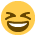Fill out The Mix's Annual User Survey for a chance to win a £200 Amazon voucher, to shape our services, and get closer to earning a badge!

# Mathematics

Posts: 1,876,324 The Mix Honorary Guru
From a strictly mathematical viewpoint it goes like: What makes 100%?
What does it mean to give MORE than 100%? Ever wonder about those
people who say they are giving more than 100%? We have all been to
those meetings where someone wants you to give over 100% How about

What makes up 100% in life?

IF
A B C D E F G H I J K L M N O P Q R S T U V W X Y Z

is represented as
1 2 3 4 5 6 7 8 9 10 11 12 13 14 15 16 17 18 19 20 21 22 23 24 25 26

Then

H-A-R-D-W-O-R-K

8+1+18+4+23+15+18+11 = 98%

and

K-N-O-W-L-E-D-G-E

11+14+15+23+12+5+4+7+5 = 96%

But,

A-T-T-I-T-U-D-E

1+20+20+9+20+21+4+5 = 100%

And,

B-U-L-L-S-H-I-T

2+21+12+12+19+8+9+20 = 103%

AND, look how far ass kissing will take you.

A-S-S-K-I-S-S-I-N-G

1+19+19+11+9+19+19+9+14+7 = 118%

So, one can then conclude with mathematical certainty that:

While Hardwork & knowledge will get you close

and Attitude will get you there,

Bullshit and Asskissing will put you over the top!
·cool!